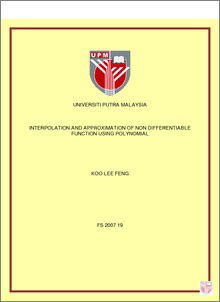# Interpolation and Approximation of Non Differentiable Function Using Polynomial

## Citation

Koo, Lee Feng (2007) Interpolation and Approximation of Non Differentiable Function Using Polynomial. Masters thesis, Universiti Putra Malaysia.

## Abstract

In any approximation problem, we concerned with a measure of closeness of polynomial ()xp to a function ()xf. Approximations are often obtained by power series expansions in which the higher order terms are dropped. One of the fundamental idea in the differential calculus is that a function can be “locally” approximated by its tangent line. If f be a function defined on an open interval I and let and Ic∈Nn∈. Suppose that the function has n-th derivative at all . Then, the polynomial Ix∈()()ccfTn, is called as Taylor polynomial of order n of function at the point c. If the function is infinitely differentiable on I, so the series is called Taylor Series of f at point c. Taylor Series is one of the fundamental idea in differential calculus. However, Taylor Series only can apply if and only if the function f be differentiated on its interval stated. A differentiable function is a continuous function. But, this is not always true that a continuous function is a differentiable function. Weierstass Approximation Theorem states that every continuous function defined on an interval []ba,can be uniformly approximated as closely as the decided function. Thus, this theorem ensure that every function can be approximate by a polynomial. Consequently, in the research, we develop a new approximation method to approximate the non differentiable function which has singularity at one point, two points and three points by using Fourier series, Lagrange Interpolation and convolution method. We will also discover the asymptotic of Lagrange Interpolation for function where λλ||)(ttf=0>λwith equidistant nodes and discover the convergence of at point t =0. Lastly, we compare the effective of Fourier series and Lagrange method in approximate the non-differentiable function.Preview
PDF
FS_2007_19.pdfView Item